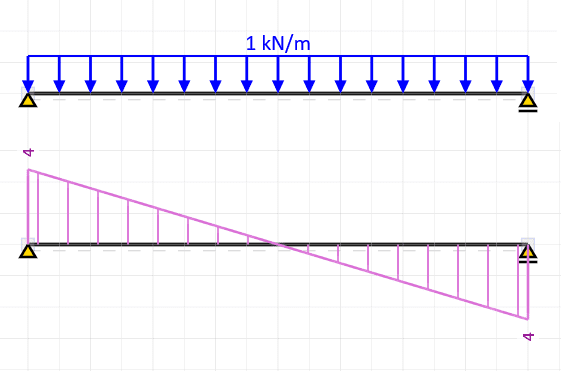# Shear Stress Distribution Along a Beam

a1234
I know that shear stress in horizontal beams has a parabolic distribution, so that the max shear stress occurs at the neutral axis. I also understand that for a beam subject to a distributed load with supports at its ends, the magnitude of the shear force is highest at the left and right ends of the beam. However, does the magnitude of the shear stress also vary along the horizontal length of the beam? If so, how?

Mentor
Do you know how to make a shear diagram? A moment diagram? And do you know the relationship between shear and moment diagrams?

What is the sign of the shear stress at the left support? At the right support? And what is the shear stress in the center (halfway between the supports) of the beam?

a1234
Shear force is positive at the left support, negative at the right support, and zero at the center of the beam. I don't fully understand how to connect the shear force along the length of the beam to its stress. I see how the shear stress varies vertically, but not horizontally along the axis of the beam.

FEAnalyst
Variation along the axis of the beam is shown on the shear force diagram:Then if you select some point along the beam axis you can calculate shear stress either using formula for average shear stress: ##\tau=\frac{V}{A}## or full formula: ##\tau=\frac{VQ}{Ib}##. In both cases V is the value obtained from shear force diagram at selected.